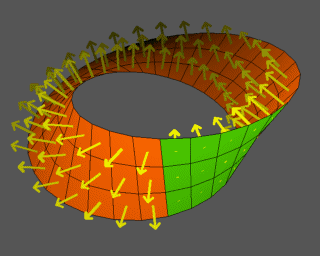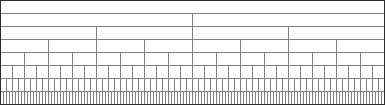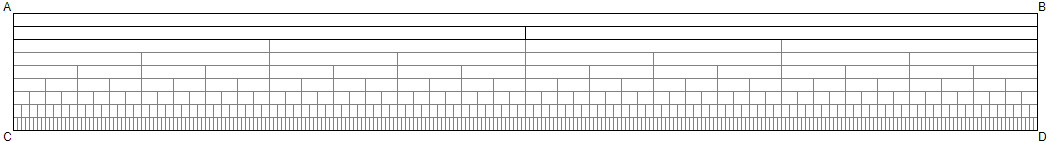Closed Loop Interval OntologyCLOSED LOOP INTERVAL ONTOLOGY        The Digital Integration of Conceptual FormTzimTzum/Kaballah | Loop definition | Home | ORIGINPlease sign in or register Email * Password * Searches selected display Universal conceptual form Aligning the vision Evolving and coalescing Why we do this Ethics / governance / science Homeostatic governance Idealized democracy Reconciliation and integration Holistic view on alternatives Definitions and alternatives Compare alternatives How do we know? How meaning is created Foundational recursive definition Spectrum of levels The closed loop ensemble contains all primary definitions Dimensions of set theory What is a number? Topology of sets How are they constructed? Primary terms Closed Loop framework Hierarchical models Euclid in digital space Foundational method Compositional semantics How it works The integrated science of mind What does it mean? Core terms Constructive elements and building blocks Preserving data under transformation In the beginning Domain and universal A design proposal Mapping the grid From other sources Foundational computation Perfecting political balance Simultaneity or sequence Concrete symbolic representation Science, philosophy and math are defined in concepts Emerging from a pointDigital geometry
Euclid in digital space

 We want to create foundational definitions for all the basic properties and objects in mathematics. We want those definitions to be defined in terms that are consistent with The Closed Loop Synthetic Dimensionality Discrete/finite mathematics Foundational concepts in mathematics, including straight lines, sets, and points

 Definition of digital geometry The Closed Loop model and the definitions it supports are defined in terms of digital or finite-state math. Our definitions of continuity are based on digital convergence, and digital increments become increasingly small We want to construct the basic definitions and concepts from traditional Euclidean geometry -- concepts such as points and line -- but we want to pursue these definitions in "constructivist" terms, such that they do not have to be "idealized" and remain solely something envisioned by the mathematician) in their mind but can in fact be "actualized". Sun, Mar 14, 2021 Reference Digital geometry deals with discrete sets (usually discrete point sets) considered to be digitized models or images of objects of the 2D or 3D Euclidean space. Simply put, digitizing is replacing an object by a discrete set of its points. The images we see on the TV screen, the raster display of a computer, or in newspapers are in fact digital images. Its main application areas are computer graphics and image analysis. Main aspects of study are: Constructing digitized representations of objects, with the emphasis on precision and efficiency (either by means of synthesis, see, for example, Bresenham's line algorithm or digital disks, or by means of digitization and subsequent processing of digital images). Study of properties of digital sets; see, for example, Pick's theorem, digital convexity, digital straightness, or digital planarity. Transforming digitized representations of objects, for example (A) into simplified shapes such as (i) skeletons, by repeated removal of simple points such that the digital topology of an image does not change, or (ii) medial axis, by calculating local maxima in a distance transform of the given digitized object representation, or (B) into modified shapes using mathematical morphology. Reconstructing "real" objects or their properties (area, length, curvature, volume, surface area, and so forth) from digital images. Study of digital curves, digital surfaces, and digital manifolds. Designing tracking algorithms for digital objects. Functions on digital space. Curve sketching, a method of drawing a curve pixel by pixel. Tracing a curve on a triangular mesh Digital geometry heavily overlaps with discrete geometry and may be considered as a part thereof.

 Numbers Wikipedia defines number as "a mathematical object used to count, measure, and label" We want to define "number" in terms mapped to the Closed Loop framework -- and connected through it to all other major mathematical and semantic definitions. Numbers are going to be defined as "intervals" -- as bounded ranges in a linear/sequential "totally ordered" structure like a row or a vector, and they are given symbolic names/labels, which we call "numerals" (0,1,2,3,4,5,6...) We are going to construct those definitions out of synthetic dimensions *** Newer idea as this project grows -- Start with the "natural numbers" Map natural numbers to objects -- like "apples" -- 1 apple, 2 apples, three apples -- first apple, second apple, third apple Integer numbers Rational numbers Sun, May 2, 2021 Reference A number is a mathematical object used to count, measure, and label. The original examples are the natural numbers 1, 2, 3, 4, and so forth. Numbers can be represented in language with number words. More universally, individual numbers can be represented by symbols, called numerals; for example, "5" is a numeral that represents the number five. As only a relatively small number of symbols can be memorized, basic numerals are commonly organized in a numeral system, which is an organized way to represent any number. The most common numeral system is the Hindu–Arabic numeral system, which allows for the representation of any number using a combination of ten fundamental numeric symbols, called digits. In addition to their use in counting and measuring, numerals are often used for labels (as with telephone numbers), for ordering (as with serial numbers), and for codes (as with ISBNs). In common usage, a numeral is not clearly distinguished from the number that it represents.